Test: Patterns - 1

# Test: Patterns - 1

Test Description

## 10 Questions MCQ Test Online MCQ Tests for Class 2 | Test: Patterns - 1

Test: Patterns - 1 for Class 2 2022 is part of Online MCQ Tests for Class 2 preparation. The Test: Patterns - 1 questions and answers have been prepared according to the Class 2 exam syllabus.The Test: Patterns - 1 MCQs are made for Class 2 2022 Exam. Find important definitions, questions, notes, meanings, examples, exercises, MCQs and online tests for Test: Patterns - 1 below.
Solutions of Test: Patterns - 1 questions in English are available as part of our Online MCQ Tests for Class 2 for Class 2 & Test: Patterns - 1 solutions in Hindi for Online MCQ Tests for Class 2 course. Download more important topics, notes, lectures and mock test series for Class 2 Exam by signing up for free. Attempt Test: Patterns - 1 | 10 questions in 20 minutes | Mock test for Class 2 preparation | Free important questions MCQ to study Online MCQ Tests for Class 2 for Class 2 Exam | Download free PDF with solutions
 1 Crore+ students have signed up on EduRev. Have you?
Test: Patterns - 1 - Question 1

### 444 is ____ 4 + 4 + 4. Which words should fill the blank?

Detailed Solution for Test: Patterns - 1 - Question 1

4 + 4 + 4 = 12

and 444 is greater than 12

So, 444 is greater than 4 + 4 + 4.

Test: Patterns - 1 - Question 2

### A 3 - digit number is formed using the clues in the box. The digit in the hundreds place is 6 more than 2. The digit in the tens place is an odd number greater than 7. The digit in the ones place is 2 less than the digit in the hundreds place. Q. What is the number?

Test: Patterns - 1 - Question 3

### Look at the numbers cards and answer questions.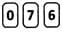What is the smallest 3-digit number that can be formed?

Test: Patterns - 1 - Question 4

Look at the numbers given and answer questions.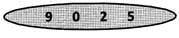Which is the smallest 3-digit even number that can be formed?

Test: Patterns - 1 - Question 5

Pandu wrote a number on the black board as shown.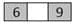If it is formed from three different digits, then which number could be placed in the gap to make it the biggest number?

Detailed Solution for Test: Patterns - 1 - Question 5

8 should be placed between 6 and 9, since 689 is the biggest number formed from three different digits 6, 8 and 9.

Test: Patterns - 1 - Question 6

60 tens is ____ 7 hundreds. Find the missing words.

Detailed Solution for Test: Patterns - 1 - Question 6

60 tens = 600 7 hundreds = 700 600 < 700 So, 60 tens < 7 hundreds

Test: Patterns - 1 - Question 7

Look at the numbers cards and answer questions.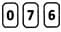What is the greatest 3-digit number that can be formed?

Test: Patterns - 1 - Question 8

Look at the numbers given and answer questions.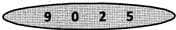Which is the greatest 3-digit odd number that can be formed?

Test: Patterns - 1 - Question 9

Ravi lost one of his numbered chickens. Use the clues given to help him find it.
Clue #1: The digit in the ones place is 5.
Clue #2: The digit in the tense place is greater than the digit in the ones place.
Clue #3: The digit in the tens place is 7 more than the digit in the hundreds place.

Detailed Solution for Test: Patterns - 1 - Question 9

Using the given clues,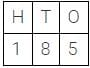the number we get is 185.

Test: Patterns - 1 - Question 10

Four students Gopi, Anand, Chintu and Akshi wrote a three digit number on their slates as shown.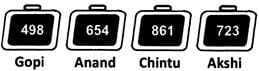Who wrote the least number?

Detailed Solution for Test: Patterns - 1 - Question 10

Gopi Since 498 is the least of the numbers written by the four students.

## Online MCQ Tests for Class 2

98 tests
 Use Code STAYHOME200 and get INR 200 additional OFF Use Coupon Code
Information about Test: Patterns - 1 Page
In this test you can find the Exam questions for Test: Patterns - 1 solved & explained in the simplest way possible. Besides giving Questions and answers for Test: Patterns - 1, EduRev gives you an ample number of Online tests for practice

98 tests